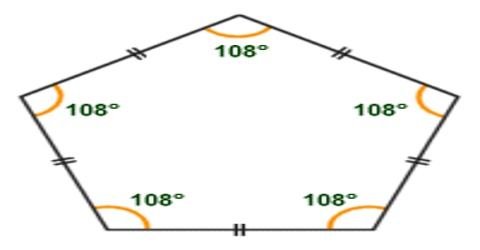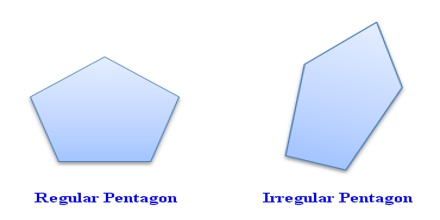Mathematic

# Pentagon: Definition with TypesA pentagon is a 5-sided Polygon. A regular pentagon has five lines of reflectional symmetry, and rotational symmetry of order 5 (through 72°, 144°, 216° and 288°). A convex pentagon has no angles pointing inwards. More precisely, no internal angles can be more than 180°. When any internal angle is greater than 180° it is concave.Properties: A regular pentagon has:

• Interior Angles of 108°
• Exterior Angles of 72°
• Area of approximately 1.7204774 × s2 (where, s = side length)

There are two types of pentagon on the basis of sides and angles:

1. Regular pentagon
2. Irregular pentagon

Regular pentagon: A pentagon which has all five sides equal in length and eventually, all the five angles are equal in measure, is known as regular pentagon. Each interior angle of a regular pentagon is 1080.

Irregular pentagon: An irregular pentagon is a pentagon which does not have all sides equal. Also, it does not have all angles equal.There are two types of pentagon on the basis of shape

1. Convex pentagon
2. Concave pentagon

Convex pentagon is a pentagon in which all five vertices are outwards. The pentagons demonstrated above are convex pentagons. A pentagon that has atleast one vertex pointing inwards is called a concave pentagon.